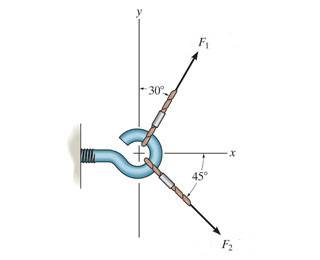# Statics Question: Finding resultant force and orientationDetermine the magnitude of the resultant force FR. Assume that F1 = 230 and F2 = 395
Express your answer to three significant figures and include the appropriate units.

Determine the direction of the resultant force FR , measured counterclockwise from the positive x axis.

I got the magnitude as 402lb which is correct, but I am having trouble finding the direction of the force.

I have the following work below

http://img5.imageshack.us/img5/1954/resultantforce.png [Broken]

So I get $\phi$=71.6 degrees

Now the first quadrant has an angle from F1 to the x axis that measures at 60 degrees. What I did was 71.6-60 degrees = 11.6 degrees and said that was the angle of the resultant force, but this answer is wrong.

Can anyone explain how to find the angle? Should I be subtracting 360 from 11.6 degrees instead since it wants the position counterclockwise?

Last edited by a moderator: